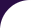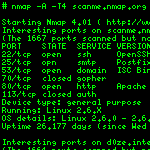Intro Reference Guide Book Install Guide Download Changelog Zenmap GUI Docs Bug Reports OS Detection Propaganda Related Projects In the Movies In the News# Library `openssl`

OpenSSL bindings.

This module is a wrapper for OpenSSL functions that provide encryption and decryption, hashing, and multiprecision integers.

The `openssl` module may not always be available. It depends on whether OpenSSL support was enabled at compile time. Scripts using the module should be made to fail gracefully using code like the following:

```if not pcall(require, "openssl") then
action = function(host, port)
stdnse.debug2("Skipping \"%s\" because OpenSSL is missing.", id)
end
end
action = action or function(host, port)
...
end```

Author:

• Sven Klemm <sven@c3d2.de>

## Functions

 bignum_add (a, b) Returns the bignum which is the result of `a+b` bignum_bin2bn (string) Converts a binary-encoded string into a bignum. bignum_bn2bin (bignum) Converts `bignum` into a binary-encoded string. bignum_bn2dec (bignum) Converts `bignum` into a decimal-encoded string. bignum_bn2hex (bignum) Converts `bignum` into a hex-encoded string. bignum_clear_bit (bignum, position) Clears the bit at `position` in `bignum`. bignum_dec2bn (string) Converts a decimal-encoded string into a bignum. bignum_div (a, b) Returns the bignums which are the result and remainder of `a/b` bignum_hex2bn (string) Converts a hex-encoded string into a bignum. bignum_is_bit_set (bignum, position) Gets the state of the bit at `position` in `bignum`. bignum_is_prime (bignum, nchecks) Checks whether `bignum` is probably prime. bignum_is_safe_prime (bignum, nchecks) Checks whether `bignum` is a safe prime. bignum_mod_exp (a, p, m) Returns the bignum which is the result of `a`^`p` mod `m`. bignum_num_bits (bignum) Returns the size of `bignum` in bits. bignum_num_bytes (bignum) Returns the size of `bignum` in bytes. bignum_pseudo_rand (bits) Returns a pseudorandom bignum. bignum_rand (bits) Returns a random bignum. bignum_set_bit (bignum, position) Sets the bit at `position` in `bignum`. decrypt (algorithm, key, iv, data, padding) Decrypt data with a given algorithm, key, and initialization vector. DES_string_to_key (data) Converts a 56-bit DES key into a 64-bit key with the correct parity. digest (algorithm, message) Returns the digest of a string using a named algorithm. encrypt (algorithm, key, iv, data, padding) Encrypt data with a given algorithm, key, and initialization vector. hmac (algorithm, key, message) Returns the message authentication code of a string using a named algorithm. md4 (message) Returns the MD4 digest of a string. md5 (message) Returns the MD5 digest of a string. rand_bytes (bytes) Returns a string containing cryptographically-strong random data. rand_pseudo_bytes (bytes) Returns a string containing pseudorandom data. rc4 (key_data) Function which generates an RC4 cipher function with the given key. rc4_options () Returns options for RC4. ripemd160 (message) Returns the RIPEMD-160 digest of a string. sha1 (message) Returns the SHA-1 digest of a string. supported_ciphers () Returns a table with the names of the supported cipher algorithms. supported_digests () Returns a table with the names of the supported digest algorithms.

## Functions

Returns the bignum which is the result of `a+b`

• a: bignum
• b: bignum

### Return value:

bignum
bignum_bin2bn (string)

Converts a binary-encoded string into a bignum.

### Parameters

• string: Binary string.

### Return value:

bignum.
bignum_bn2bin (bignum)

Converts `bignum` into a binary-encoded string.

### Parameters

• bignum: bignum to operate on.

### Return value:

Binary string.
bignum_bn2dec (bignum)

Converts `bignum` into a decimal-encoded string.

### Parameters

• bignum: bignum to operate on.

### Return value:

Decimal string.
bignum_bn2hex (bignum)

Converts `bignum` into a hex-encoded string.

### Parameters

• bignum: bignum to operate on.

### Return value:

Hex string.
bignum_clear_bit (bignum, position)

Clears the bit at `position` in `bignum`.

### Parameters

• bignum: bignum to operate on.
• position: Bit position.
bignum_dec2bn (string)

Converts a decimal-encoded string into a bignum.

### Parameters

• string: Decimal string.

### Return value:

bignum.
bignum_div (a, b)

Returns the bignums which are the result and remainder of `a/b`

• a: bignum
• b: bignum

### Return values:

1. bignum quotient
2. bignum remainder (modulo)
bignum_hex2bn (string)

Converts a hex-encoded string into a bignum.

### Parameters

• string: Hex string.

### Return value:

bignum.
bignum_is_bit_set (bignum, position)

Gets the state of the bit at `position` in `bignum`.

### Parameters

• bignum: bignum to operate on.
• position: Bit position.

### Return value:

True if the selected bit is set, false otherwise.
bignum_is_prime (bignum, nchecks)

Checks whether `bignum` is probably prime.

Performs Miller-Rabin probabilistic primality tests.

### Parameters

• bignum: bignum to check for primality
• nchecks: Number of checks to perform. Default: number of checks dependent on bitsize of bignum, with a false positive rate of at most 2^-80

### Return value:

True if the number is probably prime, false if it is composite.
bignum_is_safe_prime (bignum, nchecks)

Checks whether `bignum` is a safe prime.

A safe prime is defined as a prime p so that (p-1)/2 is also prime. Using non-safe primes in discrete logarithm cryptography like Diffie-Hellman can result in weak, easily broken key exchanges.

### Parameters

• bignum: bignum to check for primality
• nchecks: Number of checks to perform. Default: number of checks dependent on bitsize of bignum, with a false positive rate of at most 2^-80

### Return values:

1. True if the number is a safe prime, false if it is not.
2. True if the number is probably prime, false if it is composite.
bignum_mod_exp (a, p, m)

Returns the bignum which is the result of `a`^`p` mod `m`.

• a: Base.
• p: Exponent.
• m: Modulus.

### Return value:

bignum.
bignum_num_bits (bignum)

Returns the size of `bignum` in bits.

### Parameters

• bignum: bignum to operate on.

### Return value:

Size of `bignum`.
bignum_num_bytes (bignum)

Returns the size of `bignum` in bytes.

### Parameters

• bignum: bignum to operate on.

### Return value:

Size of `bignum`.
bignum_pseudo_rand (bits)

Returns a pseudorandom bignum.

### Parameters

• bits: Size of the returned bignum in bits.

### Return value:

Pseudorandom bignum.
bignum_rand (bits)

Returns a random bignum.

### Parameters

• bits: Size of the returned bignum in bits.

### Return value:

Random bignum.
bignum_set_bit (bignum, position)

Sets the bit at `position` in `bignum`.

### Parameters

• bignum: bignum to operate on.
• position: Bit position.
decrypt (algorithm, key, iv, data, padding)

Decrypt data with a given algorithm, key, and initialization vector.

### Parameters

• algorithm: Any of the strings returned by `openssl.supported_ciphers`.
• key: Key.
• iv: Initialization vector.
• data: Data to decrypt.
• padding: If true, then the final block must be padded correctly (default false).
DES_string_to_key (data)

Converts a 56-bit DES key into a 64-bit key with the correct parity.

### Parameters

• data: A 7-byte string.

### Return value:

An 8-byte string.
digest (algorithm, message)

Returns the digest of a string using a named algorithm.

### Parameters

• algorithm: Any of the strings returned by `openssl.supported_digests`.
• message: String to digest.
encrypt (algorithm, key, iv, data, padding)

Encrypt data with a given algorithm, key, and initialization vector.

### Parameters

• algorithm: Any of the strings returned by `openssl.supported_ciphers`.
• key: Key.
• iv: Initialization vector.
• data: Data to encrypt.
• padding: If true, then a partial final block will be padded and encrypted (default false).
hmac (algorithm, key, message)

Returns the message authentication code of a string using a named algorithm.

### Parameters

• algorithm: Any of the strings returned by `openssl.supported_digests`.
• key: Key.
• message: String.
md4 (message)

Returns the MD4 digest of a string.

### Parameters

• message: String to digest.

### Return value:

MD4 digest.
md5 (message)

Returns the MD5 digest of a string.

### Parameters

• message: String to digest.

### Return value:

MD5 digest.
rand_bytes (bytes)

Returns a string containing cryptographically-strong random data.

If the PRNG has not been seeded with enough randomness, this function throws an error.

### Parameters

• bytes: Length of the returned string in bytes.

### Return value:

Random string.
rand_pseudo_bytes (bytes)

Returns a string containing pseudorandom data.

No indication is given whether or not the contents of the string are cryptographically strong.

### Parameters

• bytes: Length of the returned string in bytes.

### Return value:

Pseudorandom string.
rc4 (key_data)

Function which generates an RC4 cipher function with the given key.

### Parameters

• key_data: The key for the cipher.

### Return value:

A function which performs the RC4 stream cipher on an input string and returns the result.
rc4_options ()

Returns options for RC4.

### Return value:

Option string.
ripemd160 (message)

Returns the RIPEMD-160 digest of a string.

### Parameters

• message: String to digest.

### Return value:

RIPEMD-160 digest.
sha1 (message)

Returns the SHA-1 digest of a string.

### Parameters

• message: String to digest.

### Return value:

SHA-1 digest.
supported_ciphers ()

Returns a table with the names of the supported cipher algorithms.

### Return value:

Array containing cipher names as strings.
supported_digests ()

Returns a table with the names of the supported digest algorithms.

### Return value:

Array containing digest names as strings.